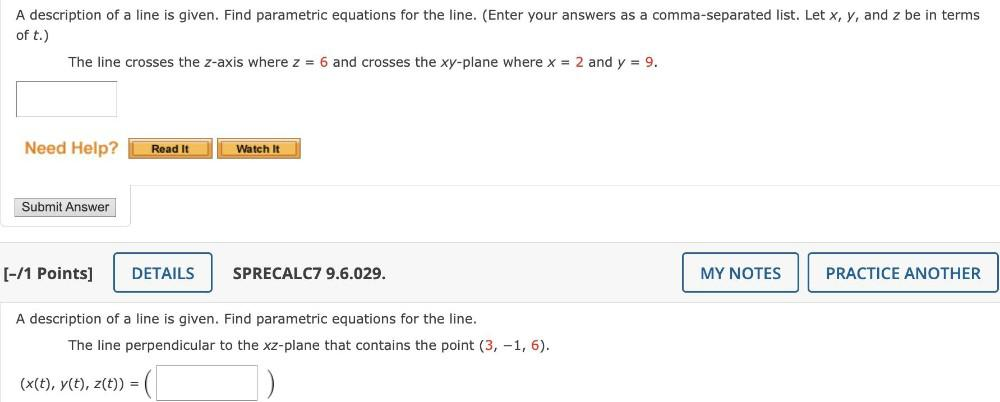Question:

# A description of a line is given. Find parametric equations for the line. (Enter your answers as a comma-separated list. Let x,A description of a line is given. Find parametric equations for the line. (Enter your answers as a comma-separated list. Let x, y, and z be in terms of t.) The line crosses the z-axis where z = 6 and crosses the xy-plane where x = 2 and y = 9. Need Help? Read It Watch It Submit Answer (-/1 Points] DETAILS SPRECALC7 9.6.029. MY NOTES PRACTICE ANOTHER A description of a line is given. Find parametric equations for the line. The line perpendicular to the xz-plane that contains the point (3, -1, 6). (x(t), y(t), z(t)) =